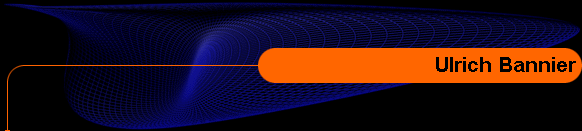Home
Physics

# Online Publications

• Rudolf Haag, Ulrich Bannier:
Comments on Mielnik's generalized(non linear) quantum mechanics.
Communications in Mathematical Physics  60, no. 1 (1978), 1–6

Abstract:  We discuss a model of non linear quantum mechanics in which the wave equation satisfies the homogeneity condition. It is argued that in this model the set of (mixed) states is a simplex.

• Ulrich Bannier:
On generally covariant quantum field theory and generalized causal and dynamical structures.
Communications in Mathematical Physics  118, no. 1 (1988), 163–170.

Abstract:  We give an example of a generally covariant quasilocal algebra associated with the massive free field. Maximal, two-sided ideals of this algebra are algebraic representatives of external metric fields. In some sense, this algebra may be regarded as a concrete realization of Ekstein's ideas of presymmetry in quantum field theory. Using ideas from our example and from usual algebraic quantum field theory, we discuss a generalized scheme, in which maximal ideals are viewed as algebraic representatives of dynamical equations or Lagrangians. The considered frame is no quantum gravity, but may lead to further insight into the relation between quantum theory and space-time geometry.

Remark:   The presented schema was successfully generalized by Romeo Brunetti, Klaus Fredenhagen and Rainer Verch

If you have Springer access, you can also read:

• Ulrich Bannier:
Intrinsic algebraic characterization of space-time structure.
International Journal of Theoretical Physics 33, no. 9 (1994), 1797-1809

Abstract:  Starting from a partially ordered set of C*-algebras R i representing algebras of observables of physical subsystems, we derive a topological Hausdorff space M as a candidate for some generalized space-time with the help of which one can define a net O→A(O), O⊆M, of algebras. This opens a way to define a physical theory without an underlying metaphysical manifold, an aspect which may be relevant for the unification of general relativity and quantum field theory.